﻿

### Some congruences modulo 2, 8 and 12 for Andrews' singular overpartitions

#### Abstract

Recently, G. E. Andrews defined combinatorial objects which he called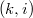$(k,i)$-singular overpartitions, overpartitions of$n$ in which no part is divisible by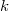$k$ and only parts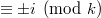$\equiv\pm i\pmod k$ may be overlined. Let the number of$(k,i)$-singular overpartitions of$n$ be\linebreak denoted by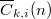$\overline{C}_{k,i}(n)$. Andrews and Chen, Hirschhorn and Sellers noted numerous congruences modulo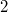$2$ for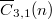$\overline{C}_{3,1}(n)$. The object of this paper is to obtain new congruences modulo$2$ for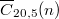$\overline{C}_{20,5}(n)$ and modulo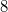$8$ and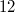$12$ for$\overline{C}_{3,1}(n)$.

DOI Code: 10.1285/i15900932v38n1p101

Keywords: singular overpartition; congruence; generating function; sums of squares

Full Text: PDF